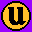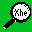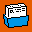# Example of debugging a shell script

To print commands and their arguments as they are executed:

```   cat example
#!/bin/sh
TEST1=result1
TEST2=result2
if [ \$TEST1 = "result2" ]
then
echo \$TEST1
fi
if [ \$TEST1 = "result1" ]
then
echo \$TEST1
fi
if [ \$test3 = "whosit" ]
then
echo fail here cos it's wrong
fi
```

This is a script called example which has an error in it; the variable \$test3 is not set so the 3rd and last test [command will fail.

Running the script produces:

```   example
result1
[: argument expected
```

The script fails and to see where the error occurred you would use the -x option like this:

```   sh -x example
TEST1=result1
TEST2=result2
+ [ result1 = result2 ]
+ [ result1 = result1 ]
+ echo result1
result1
+ [ = whosit ]
example: [: argument expected
```

The error occurs in the command [ = whosit ] which is wrong as the variable \$test3 has not been set. You can now see where to fix it.